# The Snub Dodecahedron

The snub dodecahedron is a 3D uniform polyhedron bounded by 92 polygons (12 pentagons and 80 triangles), 150 edges, and 60 vertices. It is constructed by alternating the vertices of a suitably-proportioned, non-uniform great rhombicosidodecahedron.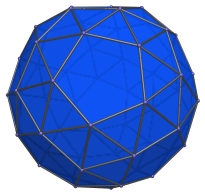The snub dodecahedron, like the snub cube, is chiral: it is not the same as its mirror image. The following images show its two enantiomorphs (mirror images):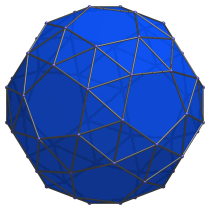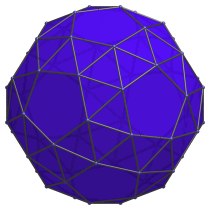The 80 triangular faces of the snub dodecahedron are of two different kinds: the first kind shares all three edges only with other triangles, and the second kind shares an edge with a pentagon, another triangle of the second kind, and a triangle of the first kind. There are 20 triangles of the first kind, shown in cyan below: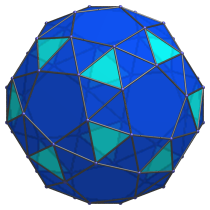These 20 triangles correspond with the faces of the regular icosahedron.

The remaining 60 triangles are of the second kind, and come in 30 pairs, corresponding with the 30 edges of the dodecahedron. The triangles in each pair share an edge with each other. These are shown in purple below: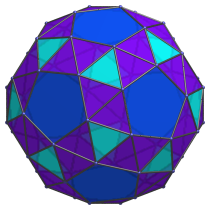## Projections

The following are projections of the snub dodecahedron from some representative viewpoints:

Projection Envelope Description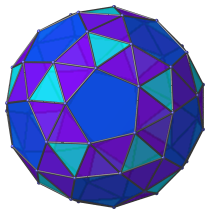Irregular icosagon

Pentagon-centered parallel projection. Note the chiral pentagonal symmetry of the projection.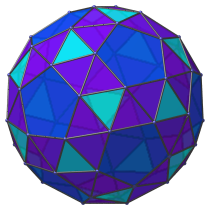Octadecagon

Parallel projection centered on a triangular face of the first kind.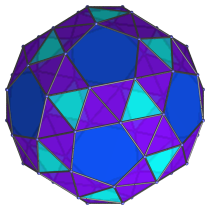Dodecagon

Parallel projection centered on an edge shared between two triangles of the second kind.

## Coordinates

The Cartesian coordinates for the snub dodecahedron, centered on the origin with edge length 2, are all even permutations of coordinates and even changes of sign of:

 ( −A, C, (Aφ+2B+C)φ ) ( −B, (A+B)φ2+C, A+(B+C)φ ) ( A+Bφ, B+C, (A+B)φ2+Cφ ) ( Aφ+B, Aφ+Bφ2+C, (A+B+C)φ ) ( −(A+B)φ, Aφ+B+C, (A+C)φ+Bφ2 )

where φ = (1+√5)/2 is the Golden Ratio, and A, B, and C are roots of the following polynomials in the indicated ranges:

 A6 − 4φ2A4 + (20φ+13)A2 − φ6 = 0, 0.6 ≤ A ≤ 0.7 B6 − 5φ2B4 + 7φ4B2 − φ4 = 0, 0.3 ≤ B ≤ 0.4 C6 + (4φ+1)C4 + 4φ4C2 − φ6 = 0, 0.7 ≤ C ≤ 0.8

Numerically, the values of A, B, and C are approximately:

• A = 0.661842049459688
• B = 0.385787422704718
• C = 0.749643316229125

Correspondingly, the coordinates are, numerically, all even permutations of coordinates and even changes of sign of:

• (−0.661842049459688, 0.749643316229125, 4.194107670504176)
• (−0.385787422704718, 3.492372881971653, 2.499007576926054)
• (1.286059211828145, 1.135430738933843, 3.955677930840437)
• (1.456670353914383, 2.830530832511964, 2.908048458676031)
• (−1.695100093578122, 2.206313670143508, 3.293835881380749)

These coordinates correspond with the alternation of a suitably-proportioned non-uniform great rhombicosidodecahedron, produced by the Minkowski sum of a dodecahedron of edge length 2A, an icosidodecahedron of edge length 2B, and an icosahedron of edge length 2C.

## Occurrences

The snub dodecahedron does not occur as cells in any uniform 4D polytopes except its own prism, the snub dodecahedron prism.

Last updated 12 Jan 2023.